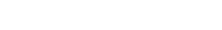# Courses Cooperative Ph. D. Program | College of Engineering | SIUCONTACT

SIU.EDU

### MATH 420-3 Abstract Algebra

Standard algebraic structures and properties. Groups: subgroups, normality and quotients, isomorphism theorems, special groups. Rings: ideals, quotient rings, special rings. Fields: extensions, finite fields, geometric constructions. Prerequisite: MATH 320 or consent of instructor.

### MATH 421-3 Linear Algebra II

Advanced study of vector spaces: Cayley-Hamilton Theorem, minimal and characteristic polynomials, eigenspaces, canonical forms, Lagrange-Sylvester Theorem, applications. Prerequisite: MATH 321 or consent of instructor.

### MATH 423-3 Combinatorics and Graph Theory

Solving discrete problems. Counting techniques, combinatorial reasoning and modeling, generating functions and recurrence relations. Graphs: definitions, examples, basic properties, applications, and algorithms. Prerequisites: MATH 223; some knowledge of programming recommended.

### MATH 435-3 Foundations for Euclidian and Non-Euclidian Geometry

Points, lines, planes, space, separations, congruence, parallelism and similarity, non-Euclidean geometries, independence of the parallel axiom. Riemannian and Bolyai-Lobachevskian geometries. Prerequisites: MATH 250; 321; MATH 320 or 350, consent of instructor.

### MATH 437-3 Differential Geometry

Curve theory, surfaces in 3-dimensional space, fundamental quadratic forms of a surface, Riemannian geometry, differential manifolds. Prerequisite: MATH 250.

### MATH 450-3 Real Analysis I

Differentiation and Riemann integration of functions of one variable. Taylor series. Improper integrals. Lebesgue measure and integration. Prerequisite: MATH 350.

### MATH 451-3 Introduction to Complex Analysis

Analytic functions, Cauchy-Riemann equations, harmonic functions, elements of conformal mapping, line integrals, Cauchy-Goursat theorem, Cauchy integral formula, power series, the residue theorem and applications. Prerequisites: MATH 223; 250.

### MATH 462-3 Engineering Numerical Analysis

Polynomial interpolation and approximations, numerical integration, differentiation, direct and iterative methods for linear systems. Numerical solutions for ODE's and PDE's. MATLAB programming required. Prerequisites: MATH 250; 305; CS 140 or 141, or consent of instructor. Not for MATH majors.

### MATH 464-3 Partial Differential Equations

Partial differential equations; Fourier series and integrals; wave equation; heat equation; Laplace equation; and Sturm-Liouville theory. Prerequisites: MATH 250, 305, and 321.

### MATH 465-3 Numerical Analysis

Error analysis, solution of nonlinear equations, interpolation, numerical differentiation and integration, numerical solution of ordinary differential equations, solution of linear systems of equations. Prerequisites: MATH 305; CS 140 or 141.

### MATH 466-3 Numerical Linear Algebra with Applications

Direct and iterative methods for linear systems, approximation of eigenvalues, solution of nonlinear systems, numerical solution of ODE and PDE boundary value problems, function approximation. Prerequisites: MATH 305; 321; CS 140 or 141.

### MATH 501-3 Differential Equations and the Fourier Analysis

Brief review of ODE. Legendre and Bessel functions. Fourier series, integrals, and transforms. Wave equation, heat equation, Laplace equation. Not for MATH majors. Prerequisite: MATH 250, MATH 305, or consent of instructor.

### MATH 502-3 Advanced Calculus for Engineers

Review of vector calculus, Green's theorem, Gauss' theorem, and Stokes' theorem. Complex analysis up to contour integrals and residue theorem. Not for MATH majors. Prerequisite: MATH 250 or consent of instructor.

### MATH 545-3 Real Analysis II

Riemann, Riemann-Stieltjes, and Lebesgue integrals. Differentiation of functions of n variables. Multiple integrals. Measure and probability. Differential forms, Stokes' Theorem. Prerequisites: MATH 321 and 450.

### MATH 552-3 Theory of Ordinary Differential Equations

Existence and uniqueness theorem, dynamical systems, stability, bifurcation theory, boundary value problems. Prerequisites: MATH 350; 421.

### MATH 555-3 Functional Analysis with Applications

Normed and Banach spaces, inner product and Hilbert spaces, Open Mapping and Closed Graph Theorem, Hahn-Banach Theorem, dual spaces and weak topology. Prerequisite: MATH 421, 450.

### MATH 563-3 Optimal Control Theory (Same as ECE 563 and ME 563)

Description of system and evaluation of its performance; dynamic programming, calculus of variations and Pontryagin's minimum principle; iterative numerical techniques. Prerequisite: MATH 305 or ECE 365 or ME 450.

### MATH 565-3 Advanced Numerical Analysis

Rigorous treatment of topics in numerical analysis including function approximation, numerical solutions to ordinary and partial differential equations. Convergence and stability of finite difference methods. Prerequisites: MATH 321; 350; 465; 466.

### Approved Engineering Science Courses for the Program Core

The courses below are taught by faculty at SIUC and will be made available at SIUE through distance education and other means. Other courses may also be taken to satisfy the Engineering Science Core requirements subject to approval of the advisor.

### ENGR 521-3 Probability and Stochastic Processes for Engineers

Axioms of probability, random variables and vectors, joint distributions, correlation, conditional statistics, sequences of random variables, stochastic convergence, central limit theorem, stochastic processes, stationarity, ergodicity, spectral analysis, and Markov processes.

### ENGR 530-3 Engineering Data Acquisition: Theory and Practice

Theory of data-acquisition and measurement systems. Criteria for selection of data acquisition hardware and software, instruments, sensors and other components for scientific and engineering experimentation. Methods for sampled data acquisition, signal conditioning, interpretation, analysis, and error estimation.

### ENGR 540-3 Design of Engineering Experiments

Planning of experiments for laboratory and field studies, factorial designs, factorial designs at two levels, fractional factorial designs, response surface methods, mixture designs. Prerequisite: MNGE 417, or MATH 483, or equivalent, or consent of instructor.

### ENGR 545-3 Advanced Numerical Methods in Engineering

Engineering applications of linear and nonlinear equations, eigenvalue problems, interpolation and approximating functions and sets of data, numerical solutions of ordinary and partial differential equations. Prerequisite: ENGR 222 or equivalent, ENGR 351 or equivalent, and Mathematics 305 or consent of instructor.